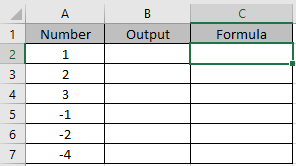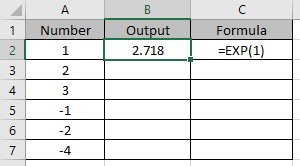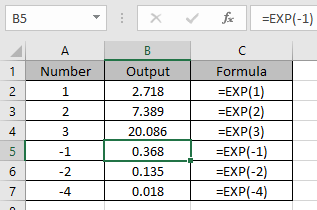# How to use the Excel EXP function

In mathematics exponential function is the e raised to the power number. The value of e is irrational and approximately equals to 2.7128.

EXP function returns the value e raised to the power number
Syntax:

=EXP(number)

Let’s understand this function using it an example.Use the formula:

=EXP(1)

The value returned here will the value of e.Use the formula in other cells to get the powers of irrational constant “e”.As you can see, the function returns the value of e and its power.
Hope you understood how to use EXP (exponential) function in Excel. Explore more articles on Excel mathematical function here. Please feel free to state your query or feedback for the above article.

Related Article:

How to use the LOG function in Excel

How to use the Excel LOG10 function

How to use the Excel LN function

Popular Articles:

How to use the VLOOKUP Function in Excel

How to use the COUNTIF function in Excel 2016

How to Use SUMIF Function in Excel

Terms and Conditions of use

The applications/code on this site are distributed as is and without warranties or liability. In no event shall the owner of the copyrights, or the authors of the applications/code be liable for any loss of profit, any problems or any damage resulting from the use or evaluation of the applications/code.